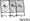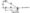# Modelling and Finite Element Analysis December 2011

Note: Answer any FIVE full questions, selecting at least TWO questions from each part.

PART-A

1 a. Write the equilibrium equation for 3-D state of stress and state the terms involved. (04 Marks)

b.  Solve the following system of equations by Gaussian elimination method :

X1 + X2 + X3 = 6

X1-x2 + 2x3 = 5

X1 + 2x2 – X3 = 2.

c. Determine the displacements of holes of the spring system shown in the figure using principle of minimum potential energy.                                                                          (08 Marks)

2 a. Explain the discretization process of a given domain based on element shapes number and size.

b.  Explain basic steps involved in FEM with the help of an example involving a structural member subjected to axial loads.

C . Why FEA is widely accepted in engineering? List various applications of FEA in engineering.

3 a.  Derive interpolation model for 2-D simplex element in global co – ordinate system.

b.  What is an interpolation function? Write the interpolation functions for:

i) 1 – D linear element ; ii)1 — Dquadistie element,

iii) 2-D linear element ; iv) 2-D quadratic element.

v) 3-D linear element.

c.   Explain “complete” and “conforming” elements.

4       a Derive shape function for 1 – D quadratic bar element in neutral co-ordinate, system.

b.   Derive shape functions for CST element in NCS.

c.   What are shape functions and write their properties, (any two).

PART-B

5  a. Derive the body force load vector for 1 – D linear bar element.

b.   Derive the Jacobian matrix for CST element starting from shape function.

c.   Derive stiffness matrix for a beam element starting from shape function.

6 a. Explain the various boundary conditions in steady state heat transfer problems with simple sketches.

b.  Derive stiffness matrix for 1 – D heat conduction problem using either functional approach or Galerkin’s approach.

c.   For the composite wall shown in the figure, derive the global stiffness matrix.

Take Ai = Aa = A3 = A7    a. The structured member shown in figure consists of two bars. An axial load of P = 200 fcN is loaded as shown. Determine the following:

i) Element stiffness matricies.

ii) Global stiffness matrix.

iv) Nodal displacements.

i)  Steel Ai = 1000 mm Ei = 200 GPa

ii)   Bronze A2 = 2000 mm2 E2 = 83 GPa.

b.  For the truss system shown, determine the nodal displacements. Assume E – 210 GPa and A = 600 mm for both elements.8  a. Determine the temperature distribution in 1 – D rectangular cross – section fin as shown in 3w figure. Assume that convection heat loss occurs from the end of the fin. Take k =Cm C0 Iw-, T= 20°C. Consider two elementsh=-Cm2°C

b.  For the cantilever beam subjected to UDL as shown in determine the deflections of the free end. Consider one element.